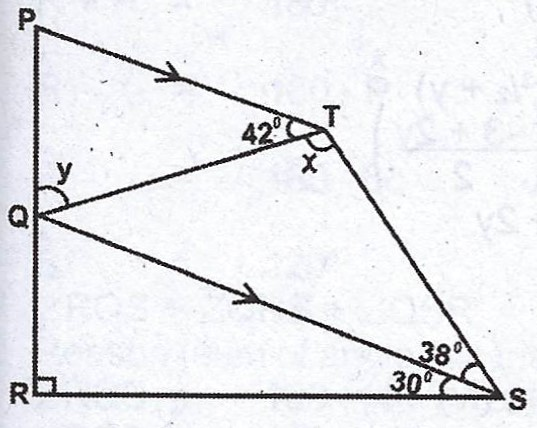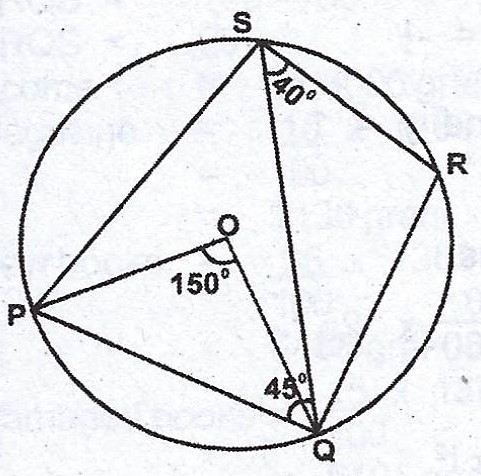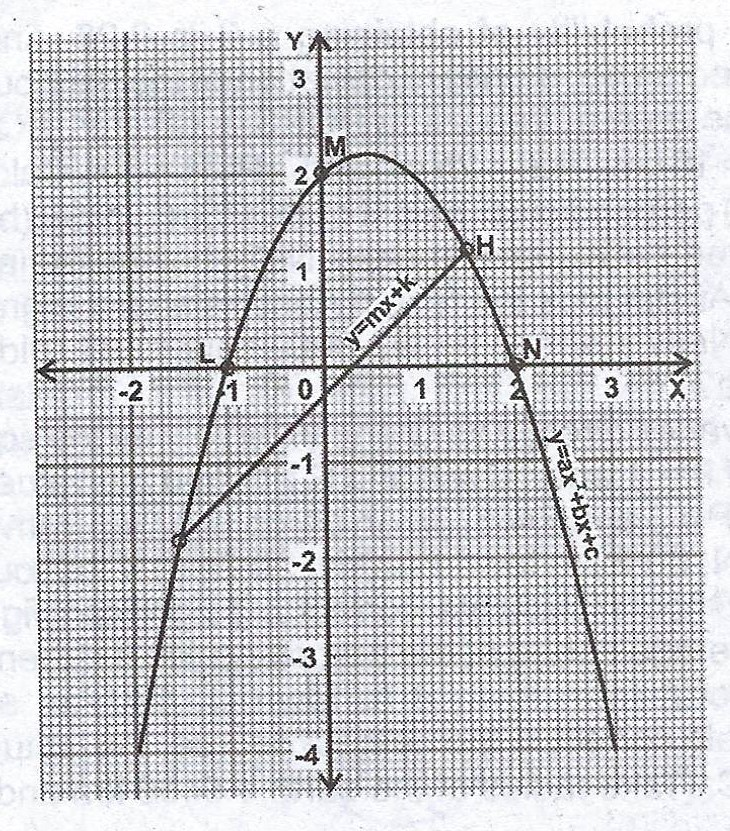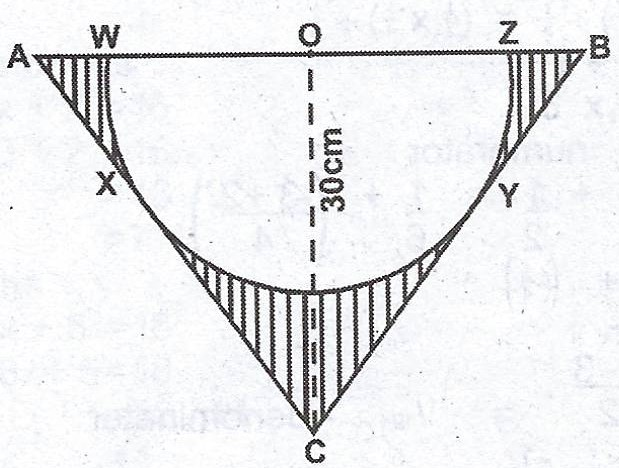### Mathematics Past Questions

3701

(a)In the diagram, PQRST is a quadrilateral. PT // QS, < PTQ = 42°, < TSQ = 38° and < QSR = 30°. If < QTS = x and < POT = y, find: (i) x ; (ii) y.

(b)In the diagram, PQRS is a circle centre O. If POQ = 150°, < QSR = 40° and < SQP = 45°, calculate < RQS.

3702

A library received \$1,300 grant. It spends 10% of the grant on magazine subscriptions, 35% on new books, 15% to repair damaged books, 30% to buy new furniture and 10% to train library staff.

(a) Represent this information on a pie chart.

(b) Calculate, correct to the nearest whole number, the percentage increase of the amount for buying books over that of new furniture.

3703

In a class of 40 students, 18 passed Mathematics, 19 passed Accounts, 16 passed Economics, 5 passed Mathematics and Accounts only, 6 Mathematics only, 9 Accounts only, 2 Accounts and Economics only. If each student offered at least one of the subjects,

(a) how many students failed in all subjects?

(b) find the percentage number that failed in at least one of Economics and Mathematics

(c) calculate the probability that a student picked at random failed in Accounts?

3704

(a) Divide $$\frac{x^{2} - 4}{x^{2} + x}$$ by $$\frac{x^{2} - 4x + 4}{x + 1}$$.

(b) The diagram below shows the graphs of $$y = ax^{2} + bx + c$$ and $$y = mx + k$$ where a, b, c and m are constants. Use the graph(s) to :

(i) find the roots of the equation $$ax^{2} + bx + c = mx + k$$;

(ii) determine the values of a, b and c using the coordinates of points L, M and N and hence write down the equation of the curve;

(iii) determine the line of symmetry of the curve $$y = ax^{2} + bx + c$$.3705

(a) Given that $$\sin x = 0.6, 0° \leq x \leq 90°$$, evaluate $$2\cos x + 3\sin x$$, leaving your answer in the form $$\frac{m}{n}$$, where m and n are integers.

(b)In the diagram, a semi-circle WXYZ with centre O is inscribed in an isosceles triangle ABC. If /AC/ = /BC/, |OC| = 30 cm and < ACB = 130°, calculate, correct to one decimal place, the (i) radius of the circle ; (ii) area oc the shaded portion. [Take $$\pi = \frac{22}{7}$$].